Скачать презентацию FINANCE 4 Bond Valuation Professeur André Farber Solvay

47b2e7d308860de6c71e4c6910e6e11c.ppt

• Количество слайдов: 28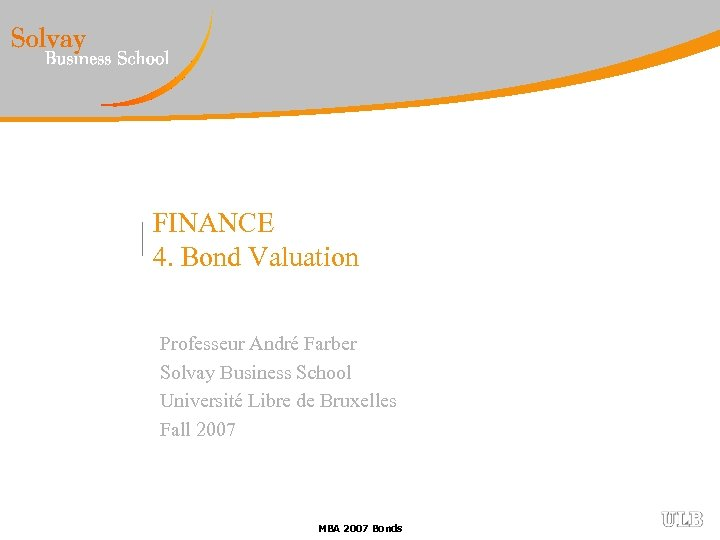FINANCE 4. Bond Valuation Professeur André Farber Solvay Business School Université Libre de Bruxelles Fall 2007 MBA 2007 Bonds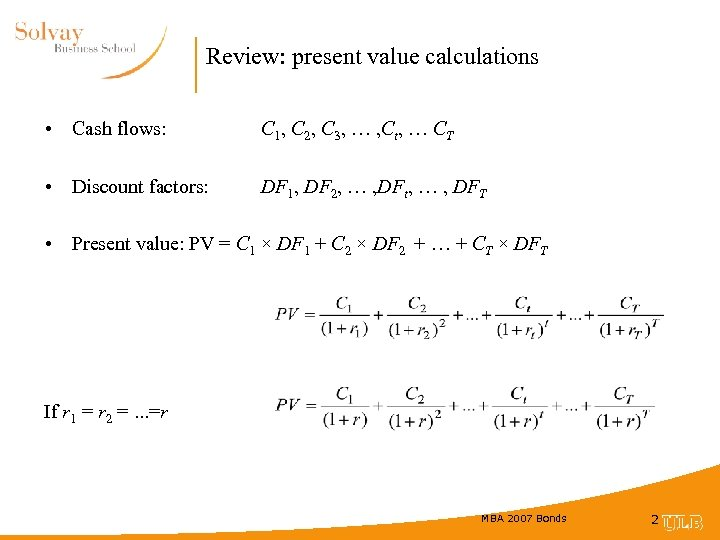Review: present value calculations • Cash flows: C 1, C 2, C 3, … , Ct, … CT • Discount factors: DF 1, DF 2, … , DFt, … , DFT • Present value: PV = C 1 × DF 1 + C 2 × DF 2 + … + CT × DFT If r 1 = r 2 =. . . =r MBA 2007 Bonds 2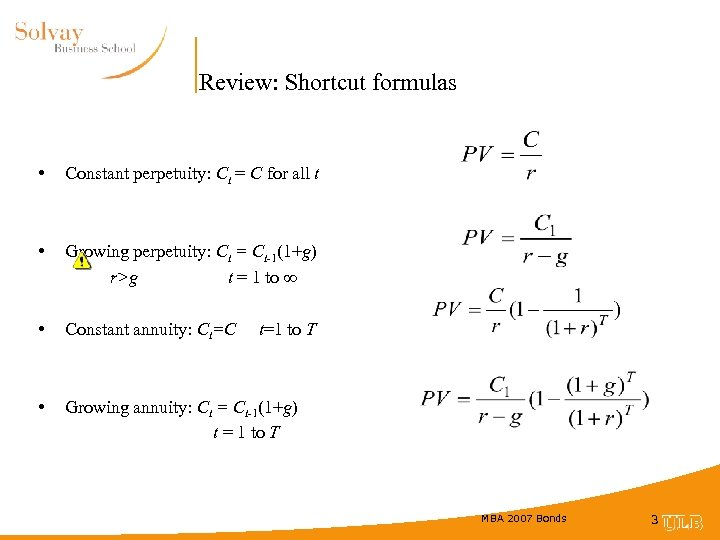Review: Shortcut formulas • Constant perpetuity: Ct = C for all t • Growing perpetuity: Ct = Ct-1(1+g) r>g t = 1 to ∞ • Constant annuity: Ct=C • Growing annuity: Ct = Ct-1(1+g) t = 1 to T t=1 to T MBA 2007 Bonds 3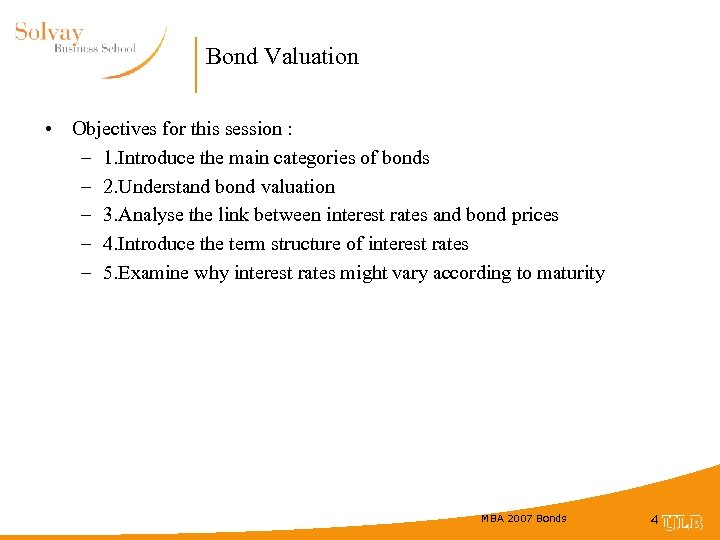Bond Valuation • Objectives for this session : – 1. Introduce the main categories of bonds – 2. Understand bond valuation – 3. Analyse the link between interest rates and bond prices – 4. Introduce the term structure of interest rates – 5. Examine why interest rates might vary according to maturity MBA 2007 Bonds 4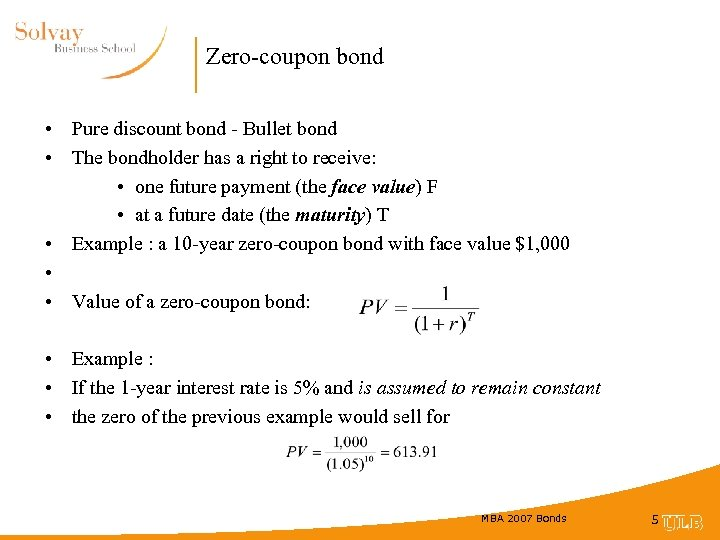Zero-coupon bond • Pure discount bond - Bullet bond • The bondholder has a right to receive: • one future payment (the face value) F • at a future date (the maturity) T • Example : a 10 -year zero-coupon bond with face value \$1, 000 • • Value of a zero-coupon bond: • Example : • If the 1 -year interest rate is 5% and is assumed to remain constant • the zero of the previous example would sell for MBA 2007 Bonds 5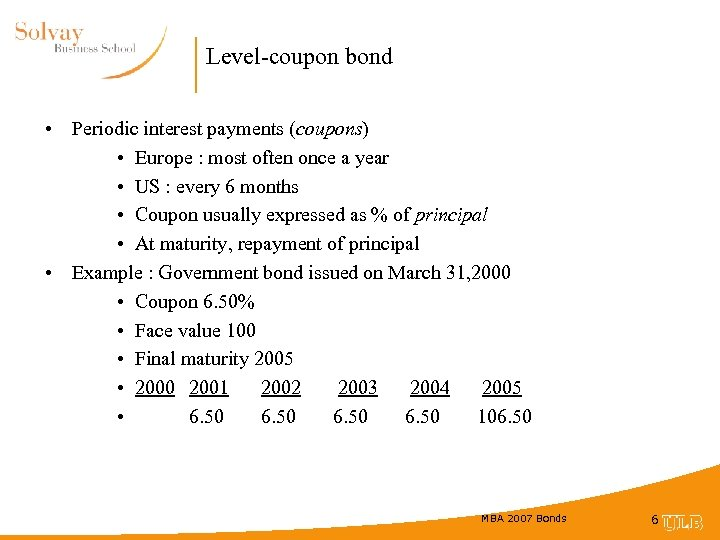Level-coupon bond • Periodic interest payments (coupons) • Europe : most often once a year • US : every 6 months • Coupon usually expressed as % of principal • At maturity, repayment of principal • Example : Government bond issued on March 31, 2000 • Coupon 6. 50% • Face value 100 • Final maturity 2005 • 2000 2001 2002 2003 2004 2005 • 6. 50 106. 50 MBA 2007 Bonds 6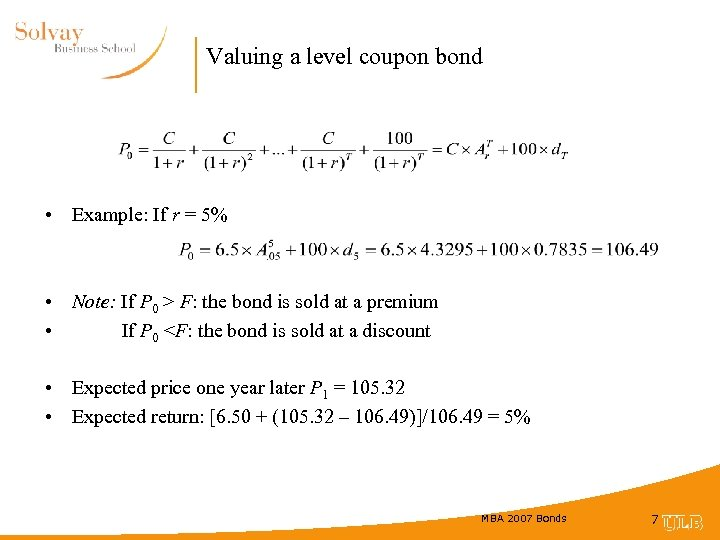Valuing a level coupon bond • Example: If r = 5% • Note: If P 0 > F: the bond is sold at a premium • If P 0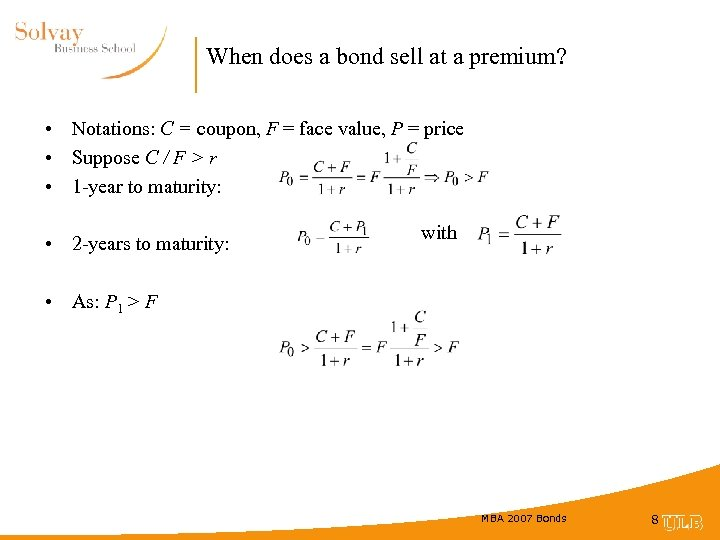When does a bond sell at a premium? • Notations: C = coupon, F = face value, P = price • Suppose C / F > r • 1 -year to maturity: • 2 -years to maturity: with • As: P 1 > F MBA 2007 Bonds 8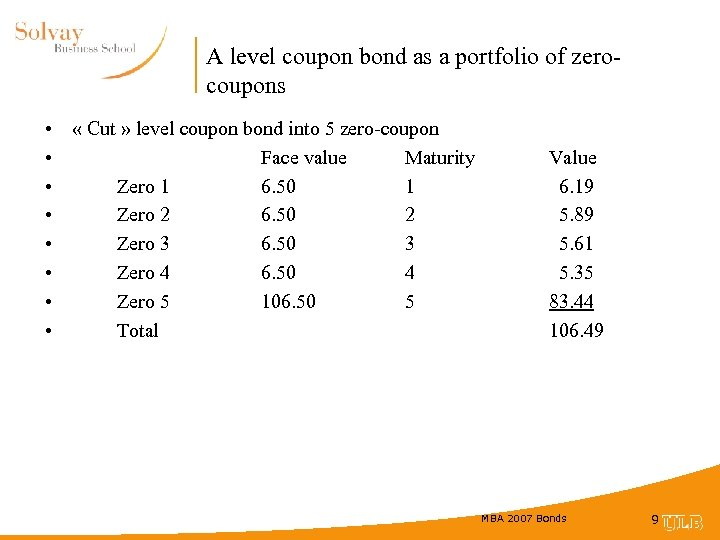A level coupon bond as a portfolio of zerocoupons • « Cut » level coupon bond into 5 zero-coupon • Face value Maturity • Zero 1 6. 50 1 • Zero 2 6. 50 2 • Zero 3 6. 50 3 • Zero 4 6. 50 4 • Zero 5 106. 50 5 • Total Value 6. 19 5. 89 5. 61 5. 35 83. 44 106. 49 MBA 2007 Bonds 9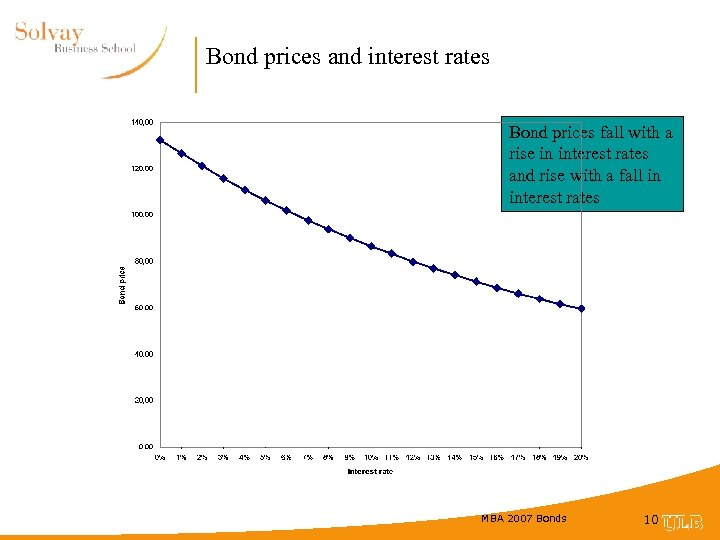Bond prices and interest rates Bond prices fall with a rise in interest rates and rise with a fall in interest rates MBA 2007 Bonds 10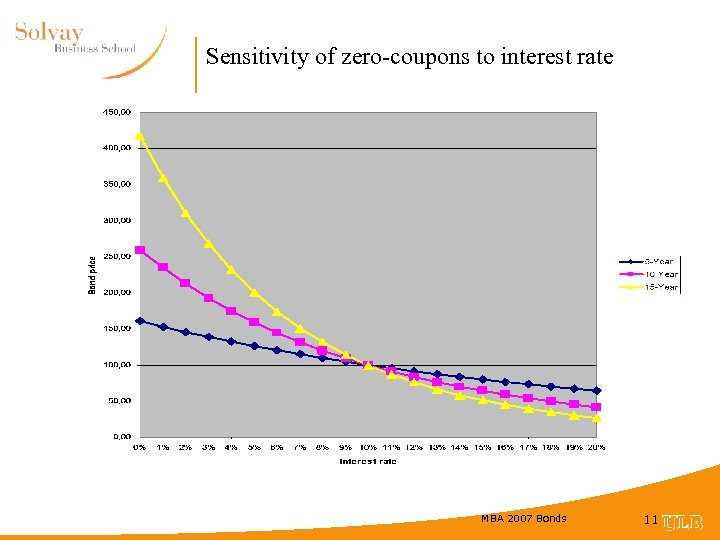Sensitivity of zero-coupons to interest rate MBA 2007 Bonds 11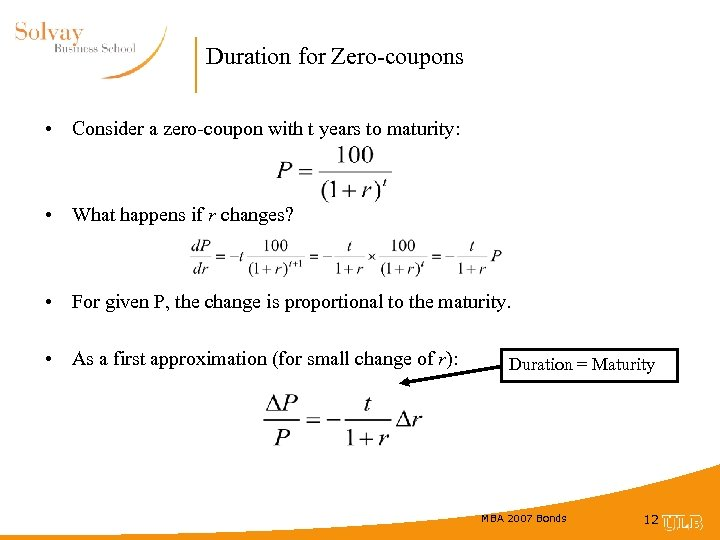Duration for Zero-coupons • Consider a zero-coupon with t years to maturity: • What happens if r changes? • For given P, the change is proportional to the maturity. • As a first approximation (for small change of r): Duration = Maturity MBA 2007 Bonds 12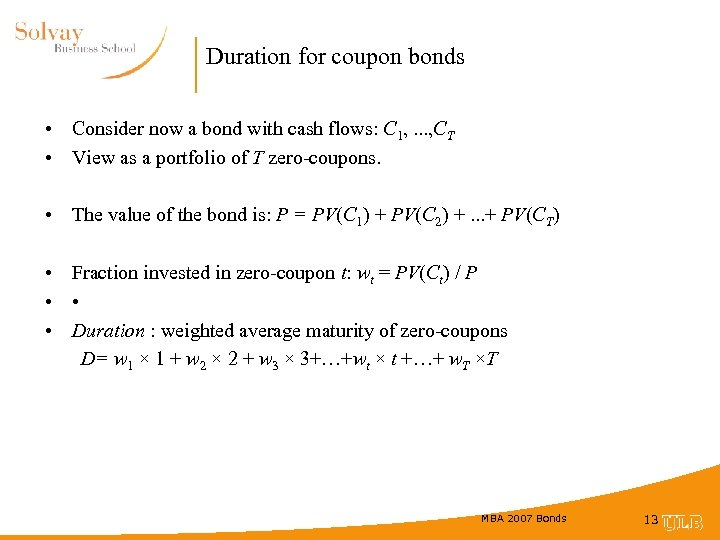Duration for coupon bonds • Consider now a bond with cash flows: C 1, . . . , CT • View as a portfolio of T zero-coupons. • The value of the bond is: P = PV(C 1) + PV(C 2) +. . . + PV(CT) • Fraction invested in zero-coupon t: wt = PV(Ct) / P • • • Duration : weighted average maturity of zero-coupons D= w 1 × 1 + w 2 × 2 + w 3 × 3+…+wt × t +…+ w. T ×T MBA 2007 Bonds 13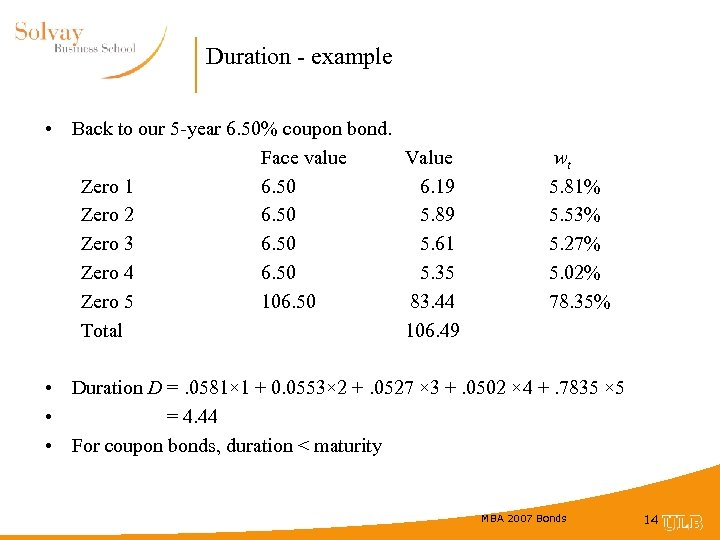Duration - example • Back to our 5 -year 6. 50% coupon bond. Face value Value Zero 1 6. 50 6. 19 Zero 2 6. 50 5. 89 Zero 3 6. 50 5. 61 Zero 4 6. 50 5. 35 Zero 5 106. 50 83. 44 Total 106. 49 wt 5. 81% 5. 53% 5. 27% 5. 02% 78. 35% • Duration D =. 0581× 1 + 0. 0553× 2 +. 0527 × 3 +. 0502 × 4 +. 7835 × 5 • = 4. 44 • For coupon bonds, duration < maturity MBA 2007 Bonds 14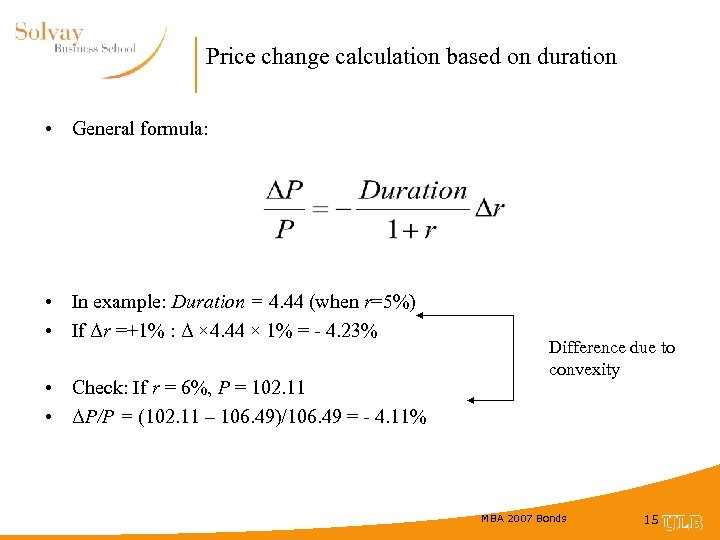Price change calculation based on duration • General formula: • In example: Duration = 4. 44 (when r=5%) • If Δr =+1% : Δ × 4. 44 × 1% = - 4. 23% • Check: If r = 6%, P = 102. 11 • ΔP/P = (102. 11 – 106. 49)/106. 49 = - 4. 11% Difference due to convexity MBA 2007 Bonds 15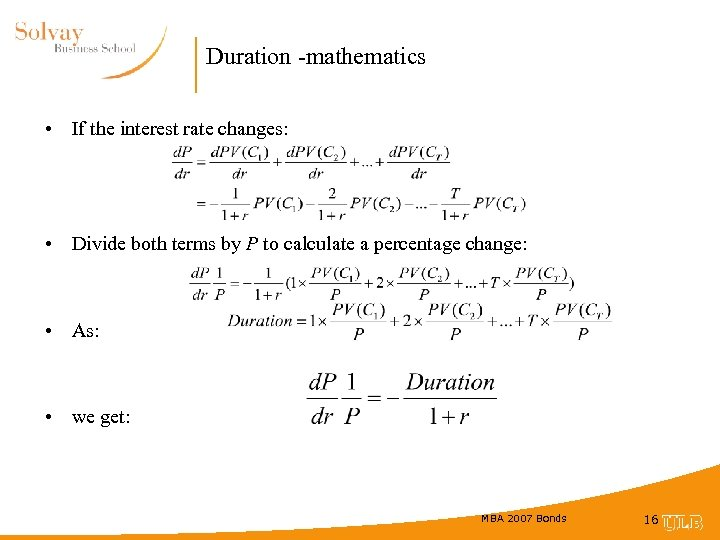Duration -mathematics • If the interest rate changes: • Divide both terms by P to calculate a percentage change: • As: • we get: MBA 2007 Bonds 16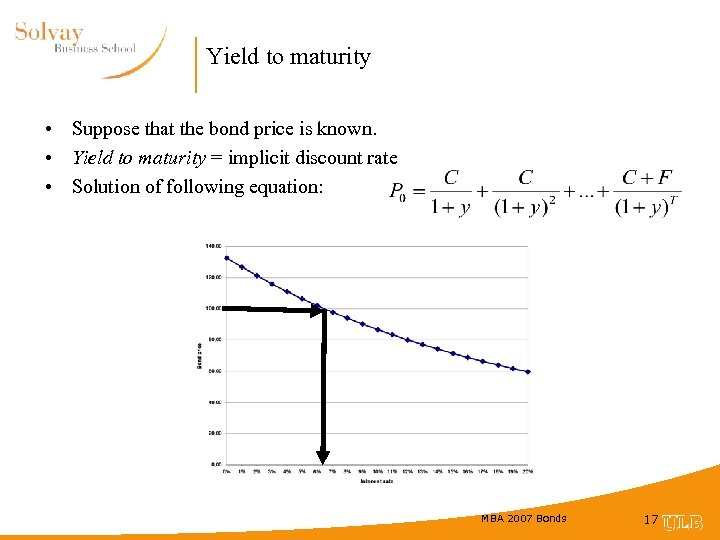Yield to maturity • Suppose that the bond price is known. • Yield to maturity = implicit discount rate • Solution of following equation: MBA 2007 Bonds 17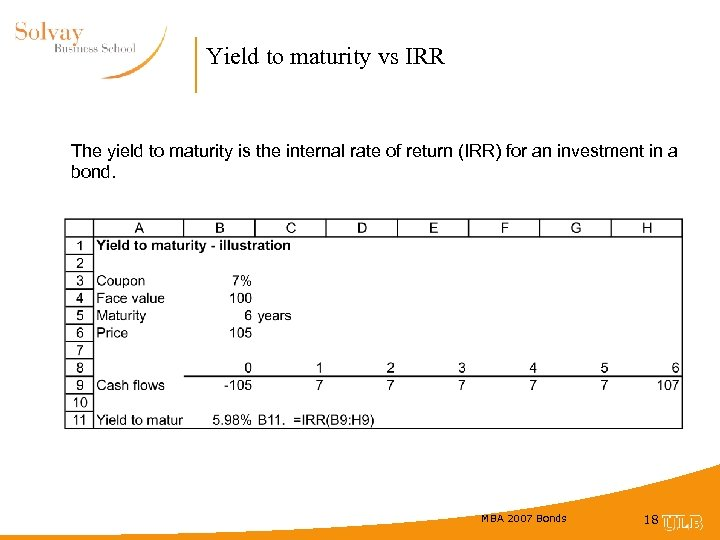Yield to maturity vs IRR The yield to maturity is the internal rate of return (IRR) for an investment in a bond. MBA 2007 Bonds 18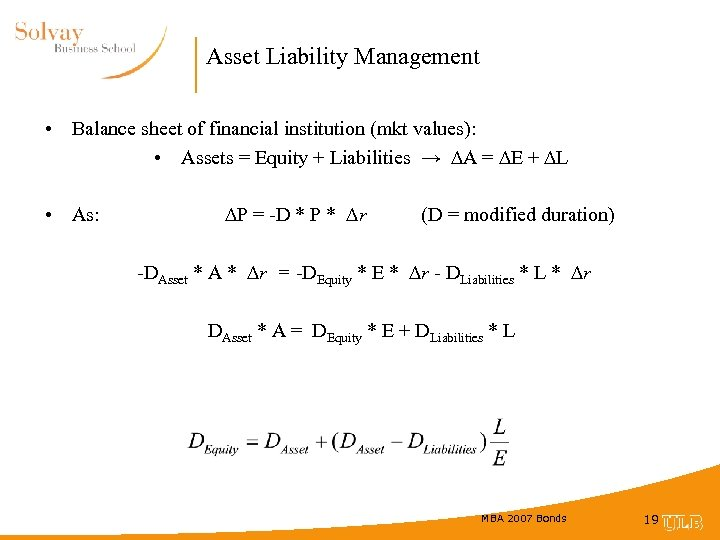Asset Liability Management • Balance sheet of financial institution (mkt values): • Assets = Equity + Liabilities → ∆A = ∆E + ∆L • As: ∆P = -D * P * ∆r (D = modified duration) -DAsset * A * ∆r = -DEquity * E * ∆r - DLiabilities * L * ∆r DAsset * A = DEquity * E + DLiabilities * L MBA 2007 Bonds 19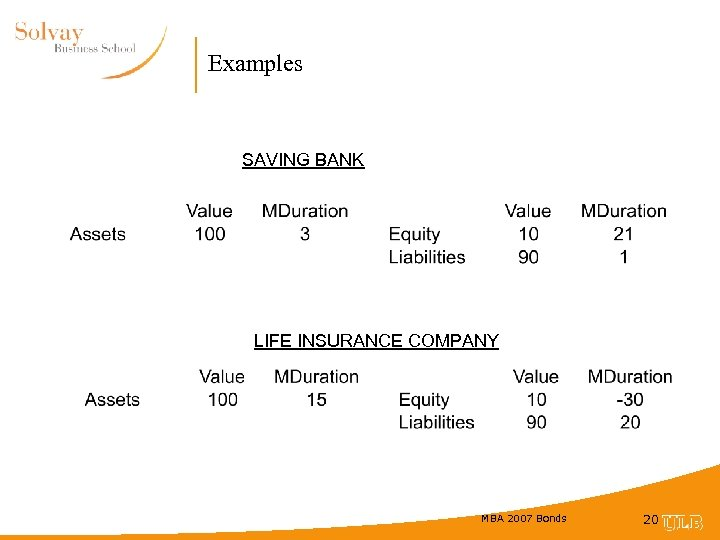Examples SAVING BANK LIFE INSURANCE COMPANY MBA 2007 Bonds 20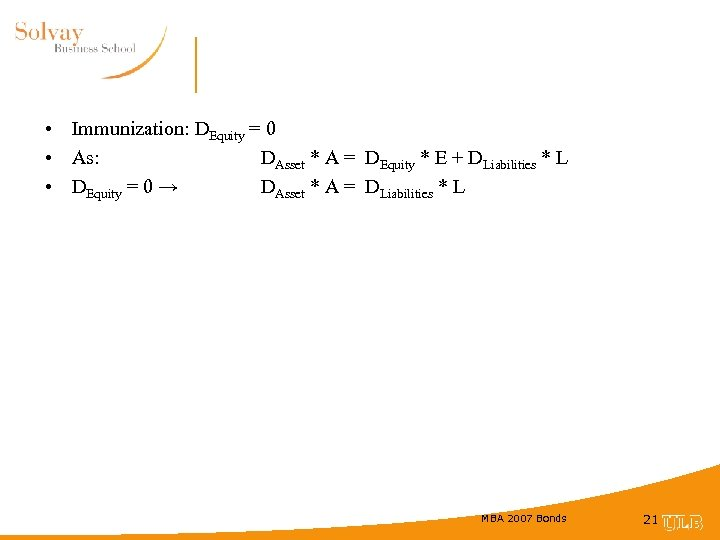• Immunization: DEquity = 0 • As: DAsset * A = DEquity * E + DLiabilities * L • DEquity = 0 → DAsset * A = DLiabilities * L MBA 2007 Bonds 21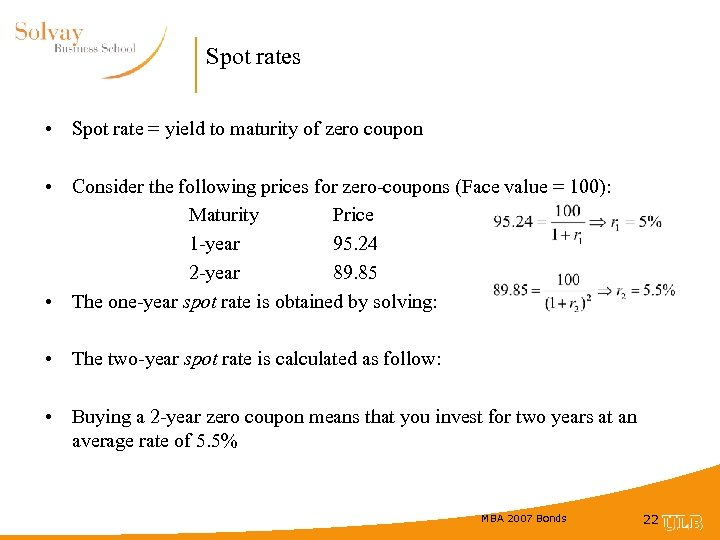Spot rates • Spot rate = yield to maturity of zero coupon • Consider the following prices for zero-coupons (Face value = 100): Maturity Price 1 -year 95. 24 2 -year 89. 85 • The one-year spot rate is obtained by solving: • The two-year spot rate is calculated as follow: • Buying a 2 -year zero coupon means that you invest for two years at an average rate of 5. 5% MBA 2007 Bonds 22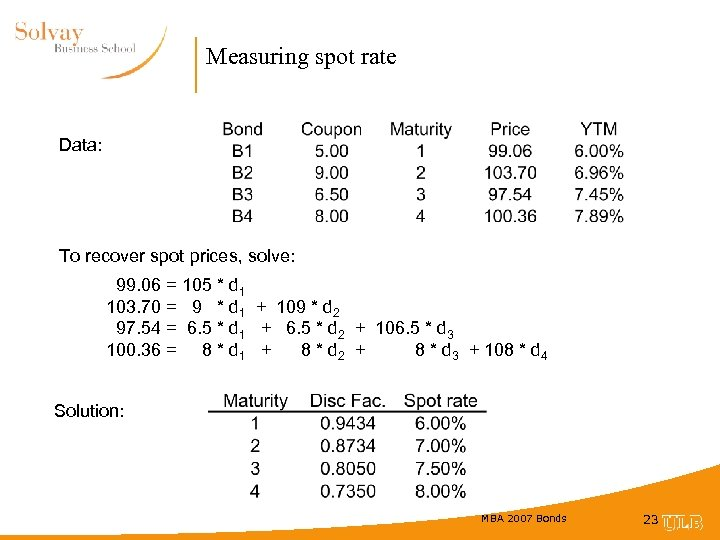Measuring spot rate Data: To recover spot prices, solve: 99. 06 = 105 * d 1 103. 70 = 9 * d 1 + 109 * d 2 97. 54 = 6. 5 * d 1 + 6. 5 * d 2 + 106. 5 * d 3 100. 36 = 8 * d 1 + 8 * d 2 + 8 * d 3 + 108 * d 4 Solution: MBA 2007 Bonds 23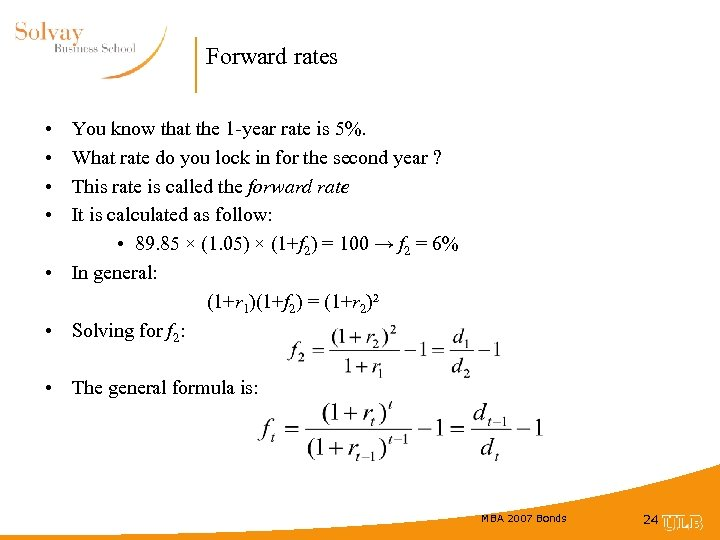Forward rates • • You know that the 1 -year rate is 5%. What rate do you lock in for the second year ? This rate is called the forward rate It is calculated as follow: • 89. 85 × (1. 05) × (1+f 2) = 100 → f 2 = 6% • In general: (1+r 1)(1+f 2) = (1+r 2)² • Solving for f 2: • The general formula is: MBA 2007 Bonds 24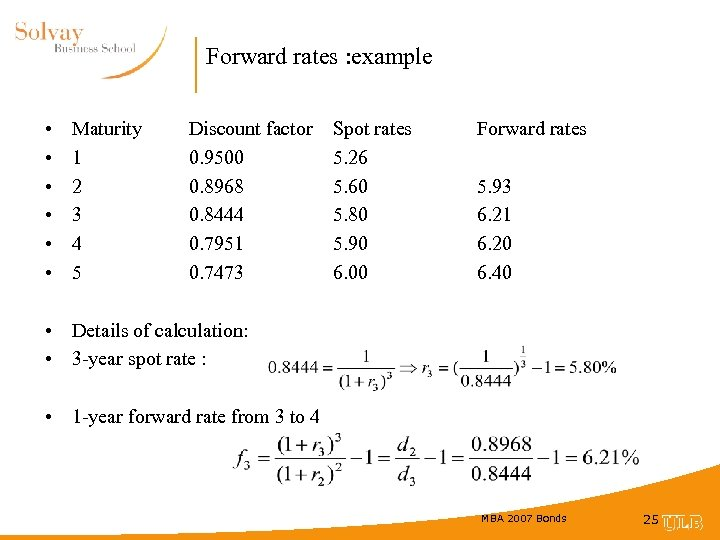Forward rates : example • • • Maturity 1 2 3 4 5 Discount factor 0. 9500 0. 8968 0. 8444 0. 7951 0. 7473 Spot rates 5. 26 5. 60 5. 80 5. 90 6. 00 Forward rates 5. 93 6. 21 6. 20 6. 40 • Details of calculation: • 3 -year spot rate : • 1 -year forward rate from 3 to 4 MBA 2007 Bonds 25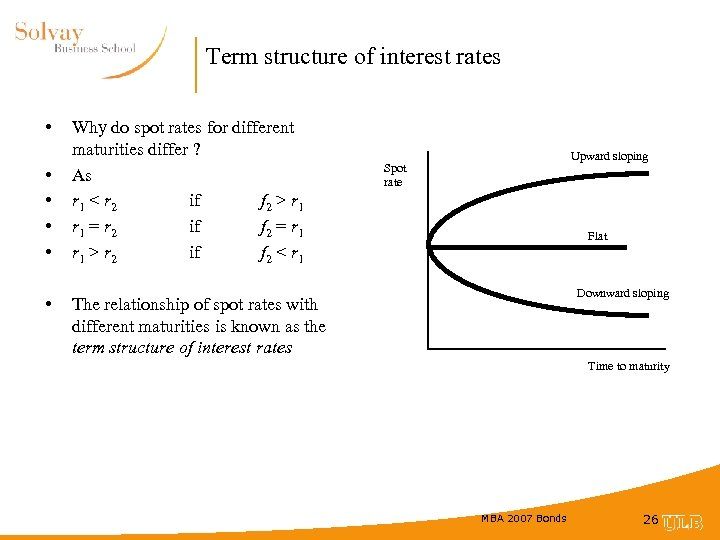Term structure of interest rates • • • Why do spot rates for different maturities differ ? As r 1 < r 2 if f 2 > r 1 = r 2 if f 2 = r 1 > r 2 if f 2 < r 1 Upward sloping Spot rate Flat Downward sloping The relationship of spot rates with different maturities is known as the term structure of interest rates Time to maturity MBA 2007 Bonds 26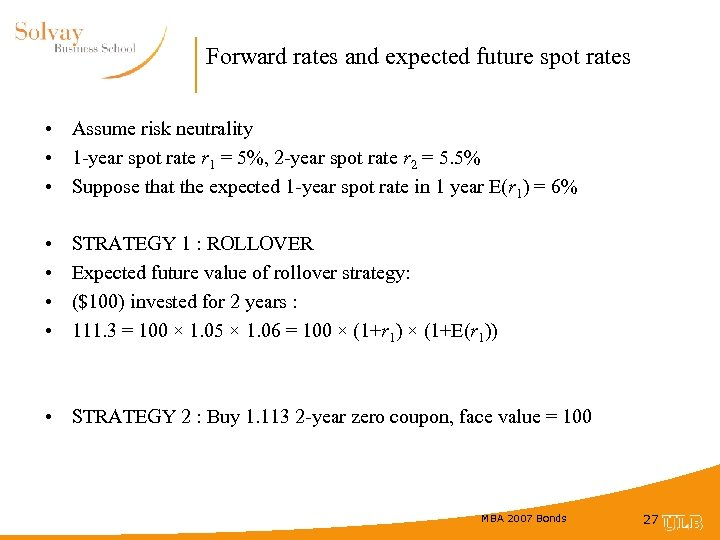Forward rates and expected future spot rates • Assume risk neutrality • 1 -year spot rate r 1 = 5%, 2 -year spot rate r 2 = 5. 5% • Suppose that the expected 1 -year spot rate in 1 year E(r 1) = 6% • • STRATEGY 1 : ROLLOVER Expected future value of rollover strategy: (\$100) invested for 2 years : 111. 3 = 100 × 1. 05 × 1. 06 = 100 × (1+r 1) × (1+E(r 1)) • STRATEGY 2 : Buy 1. 113 2 -year zero coupon, face value = 100 MBA 2007 Bonds 27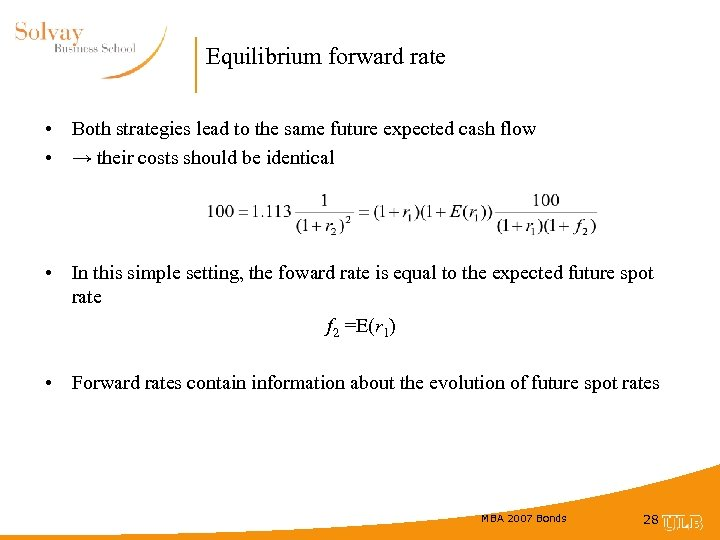Equilibrium forward rate • Both strategies lead to the same future expected cash flow • → their costs should be identical • In this simple setting, the foward rate is equal to the expected future spot rate f 2 =E(r 1) • Forward rates contain information about the evolution of future spot rates MBA 2007 Bonds 28